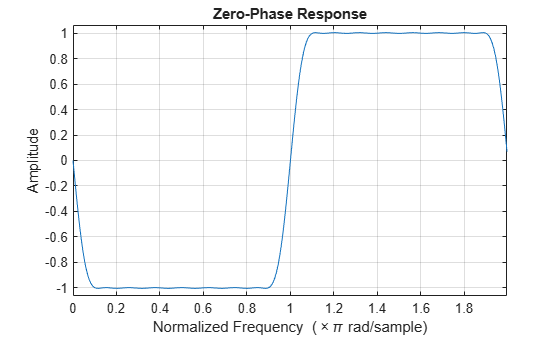# firtype

Determine type (1-4) of linear phase FIR filter System object

## Syntax

``type = firtype(sysobj)``

## Description

example

````type = firtype(sysobj)` determines the type (1 through 4) of the FIR filter System object™For more input options, see `firtype` in Signal Processing Toolbox™.```

## Examples

collapse all

Design a Hilbert transformer of order 30 with a transition width of 0.2π rad/sample. Use least-squares minimization to obtain an equiripple linear-phase FIR filter. Plot the zero-phase response in the interval [–π,π).

```d = fdesign.hilbert('N,TW',30,0.2); Hd = design(d,'equiripple',SystemObject=true); zerophase(Hd,'whole')```The impulse response of this even-order type-3 filter is antisymmetric.

`impz(Hd)``ftype = firtype(Hd)`
```ftype = 3 ```

Design a minimum-order Hilbert transformer that has a sample rate of 1 kHz. Specify the width of the transition region as 10 Hz and the passband ripple as 1 dB. Display the zero-phase response of the filter.

```fs = 1e3; d = fdesign.hilbert('TW,Ap',10,1,fs); hd = design(d,'equiripple',SystemObject=true); zerophase(hd,-fs/2:0.1:fs/2,fs)```## Input Arguments

collapse all

Input FIR filter with real and linear phase, specified as one of the following filter System objects:

To check if a filter has linear phase, use the `islinphase` function. To check if a filter has real coefficients, use the `isreal` function.

## Output Arguments

collapse all

FIR filter type, defined as one of the following:

`1` –– Type 1 filter with even order symmetric coefficients.

`2` –– Type 2 filter with odd order symmetric coefficients.

`3` –– Type 3 filter with even order antisymmetric coefficients.

`4` –– Type 4 filter with odd order antisymmetric coefficients.

## Version History

Introduced in R2013a

expand all